PHY294H - Lecture 43

The ray analysis we used in geometrical optics did not achnowledge the wave nature of light. The light traveled in straight lines, except for reflection and refraction. The wave nature of light is evident in two types of phenomena, interference and diffraction. These effects are most evident when the wavelength of light is close to the size of the object or hole that we are interested in. Even when the size of an object is considerably larger than the wavelength of light we can see interference and diffraction effects by use a screen which is a long way from the scattering event or events.

Phase differences and interference

Waves from two different sources interfere. You have probably observed this with water waves at the beach and in ripples on ripple tanks. In order for interference to occur, in a well defined way, the light from the two sources needs to have a well defined relative phase and it is simplest if they have the same polarisation. In all interference experiments we need to compare the phase of the waves arriving from two or more sources. If the waves are in phase they add constructively, while if they are out of phase they cancel each other ( they add destructively). Often we shall calculate the phase difference,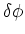, between the waves coming from two sources. In that case we have the following conditions: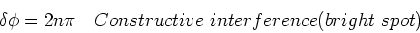(1)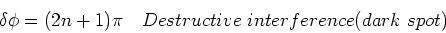(2)

where n is an integer. There are many ways to change the phase difference between the two waves, including differences in path-length, differences in refractive index experienced by the two rays, differences in phases of the two sources.

Young's double slit experiment

Young's double slit experiment consists of a coherent light source incident upon two slits. The light coming out of the slits is like the light coming from two pencil-like sources of light, which are in phase. The light arriving at different locations on a screen have different path lengths. The distance from a point on the screen to one of the slits is l1, while the distance from a point on the screen to the other slit is l2. Now we need to relate distance to phase. The relation is that one wavelength corresponds to a phase of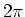. The phase difference between the two light waves arriving from the two sources is then,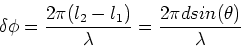(3)

[Aside: The equation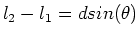is a small angle approximation. The complete relation goes as follows: Consider the three lengths: l1 from the first(upper) slit to a position P on the screen; l from a point midpoint between the slits to point P; l2 from the second(lower) slit to the screen. The line l makes an anglewith the horizontal. Consider the triangle formed by l, l1 and the vertical distance d/2 between the center point between the slits and the upper slit. The angle between l and the vertical in this triangle is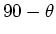. Using the cosine rule on this triangle yields,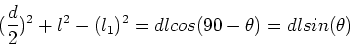(4)

Similarly, the lower triangle yields,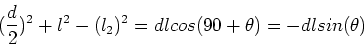(5)

Subtracting the second equation from the first gives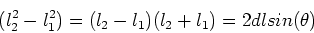(6)

From this it is seen that the pathlength difference is given by,(7)

where the last expression involves the approximation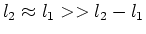.]

The angles at which maxima are located are found from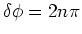, which gives,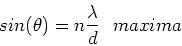(8)

and for minima,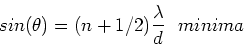(9)

where n is an integer.

The intensity pattern

The pathlength argument above only gives the location of the maxima and minima. Now we want to find the intensity pattern that we see on the screen. To find the intensity at a point on the screen, we calculate,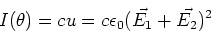(10)

We shall take the waves to have to have the same polarization and only differ by a phase given by Eq. (3). We thus write,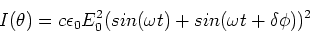(11)

Use the trigonometric identity,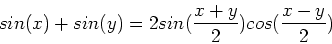(12)

So that,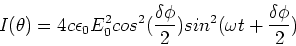(13)

This may be written as,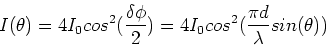(14)

where I0 is the intensity due to a single source.

Interference due to films and air gaps

When light is incident on any interface, some reflection occurs, even if the material is transparent (e.g. reflections off glass). The light which reflects off the top surface may interfere with the light which reflects off the bottom surface of a thin film. This is the origin of color in thin soap films, oil films and in narrow air gaps. These films look colored due to the fact that the different colors have different wavelengths, leading to different locations for the maxima and minima of the interference patterns. We shall illustrate the phenomena for monochromatic light, as we did for Young's experiment.

Interference due to an air gap

Consider two flat plates of glass which make an air wedge. If we illuminate this wedge with monochromatic light, an interference pattern is produced in which the light reflected from the top surface of the air wedge interferes the light reflected from the bottom surface of the air wedge. If the air wedge has a small angle, then the size of the air gap is given by,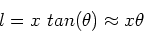(15)

The light which reflects from the bottom surface of the air wedge travels an additional distance 2l(for near normal incidence), which leads to a phase difference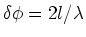. To determine the conditions constructive and destructive interference, we need to know one other important fact:

- When light reflects from a surface where n1>n2, there is no phase change of the reflected light.

- When light reflects from a surface where n1<n2, there is a phase change of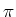of the reflected light.

This is analogous to what happens with waves on strings where the density of the string replaces the refractive index. Given this information, we find,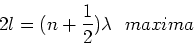(16)

and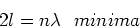(17)